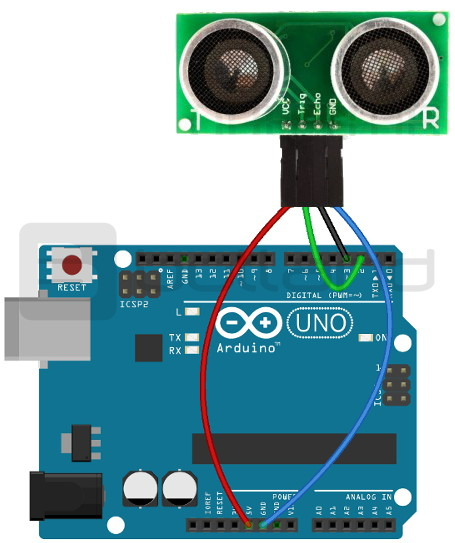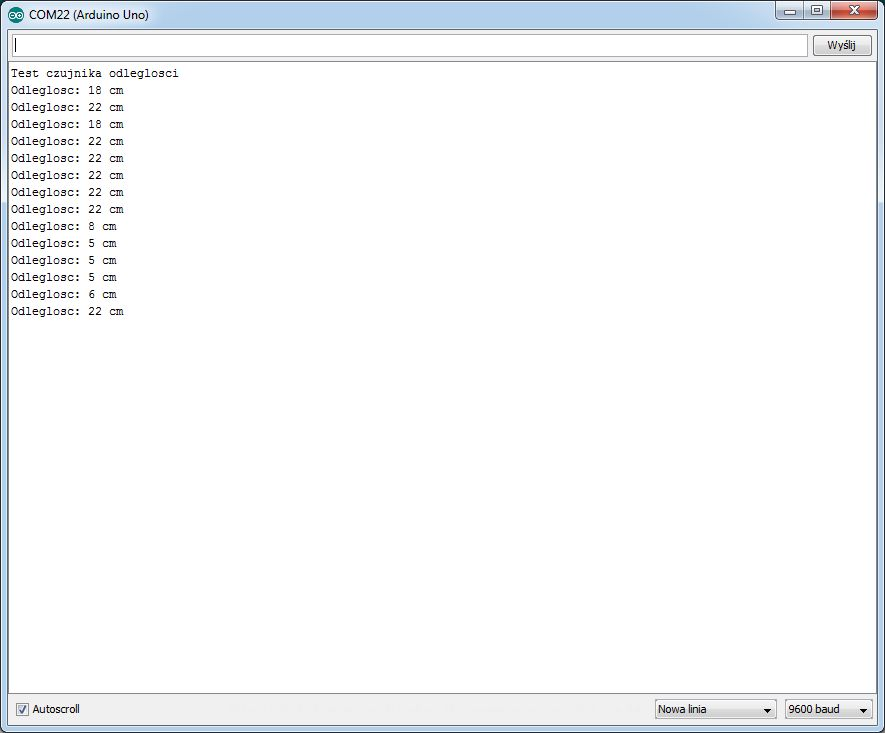# Distance measurement using Arduino and HC-SR04 sensor

## Distance measurement with Arduino sensor HC-SR04 US-015

Presents sensors provide distance measurement using ultrasound. The sensor sends out a wave, which after reflection from the obstacle, it returns to him. Computing the time between transmission and reception of the pulse, we can determine the distance to the object.

#### Connect the sensor with Arduino:

The sensor using the Arduino you should connect the layout as follows:

Pin BH1750Pin Arduino
VCC5 V
GNDGND
TRIG2
ECHO

3Connection diagram of ultrasonic sensor with Arduino Uno.

#### Program for Arduino

In the example, we used the following code:

```int Trig = 2; //pin 2 of Arduino is connected to the contact sensor Trigger
int Echo = 3; //Arduino pin 3 is connected to the contact sensor Echo
int CM; //distance in cm
long TIME; //duration of the reverse pulse in the uS

void setup()
{
Serial.begin(9600); //inicjalizaja monitor serial port
pinMode(Trig, OUTPUT); //setting Arduino pin 2 as output
pinMode(Echo, INPUT); //setting pin 3 in Arduino as input

Serial.println("Test of spacing");
}

void loop()
{
pomiar_odleglosci(); //measure the distance
Serial.print("Distance: "); //display results on the screen in a loop every 200 MS
Serial.print(CM);
Serial.println(" cm");
delay(200);
}

void pomiar_odleglosci ()
{
digitalWrite(Trig, LOW); //setting the high status on 2 uS pulse inicjalizujacy - see the documentation
delayMicroseconds(2);
digitalWrite(Trig, HIGH); //setting the high position for 10 uS pulse inicjalizujacy - see the documentation
delayMicroseconds(15);
digitalWrite(Trig, LOW);
digitalWrite(Echo, HIGH);
TIME = pulseIn(Echo, HIGH);
CM = DURATION / 58; //width of the reflected pulse in the uS divided by 58 is the distance in centimeters - see the documentation
}
```

To calculate the distance to the object, the response time is common to 58 (according to the documentation). Why so much? This value is derived by the formula:

TIME / [1/(0.34 / 2 )/10]

• the value in square brackets is equal to 58:
• 0.34 - wave velocity in m/MS (340 m/sec) divided by 2 (since the wave has come a long way in the two sides).
• In addition, all safely by 10 to change millimetres to centimeters

The result of the program can be viewed on the serial monitor:A screenshot of the serial monitor.